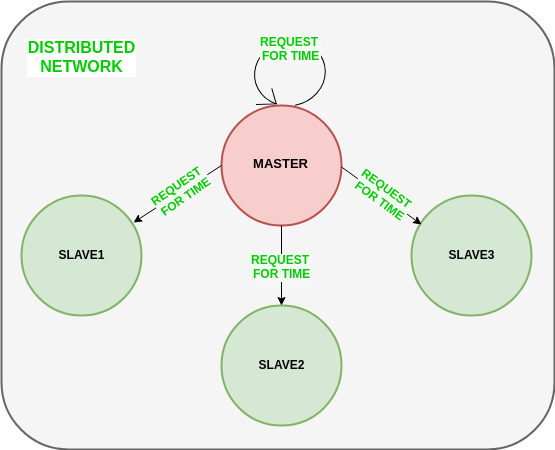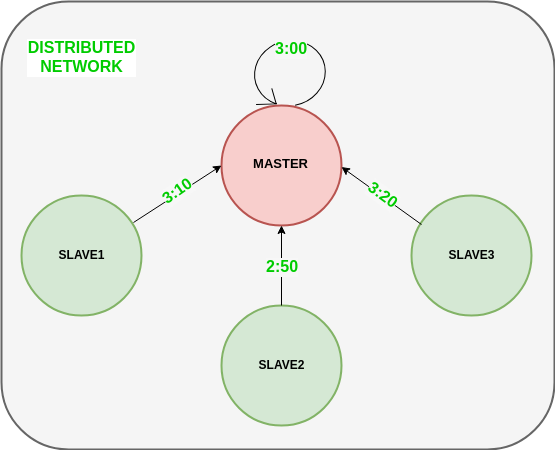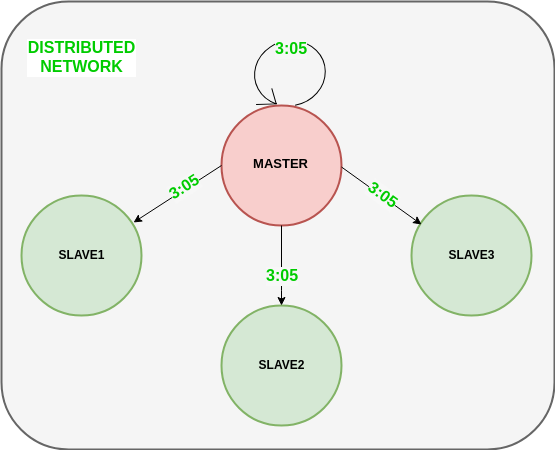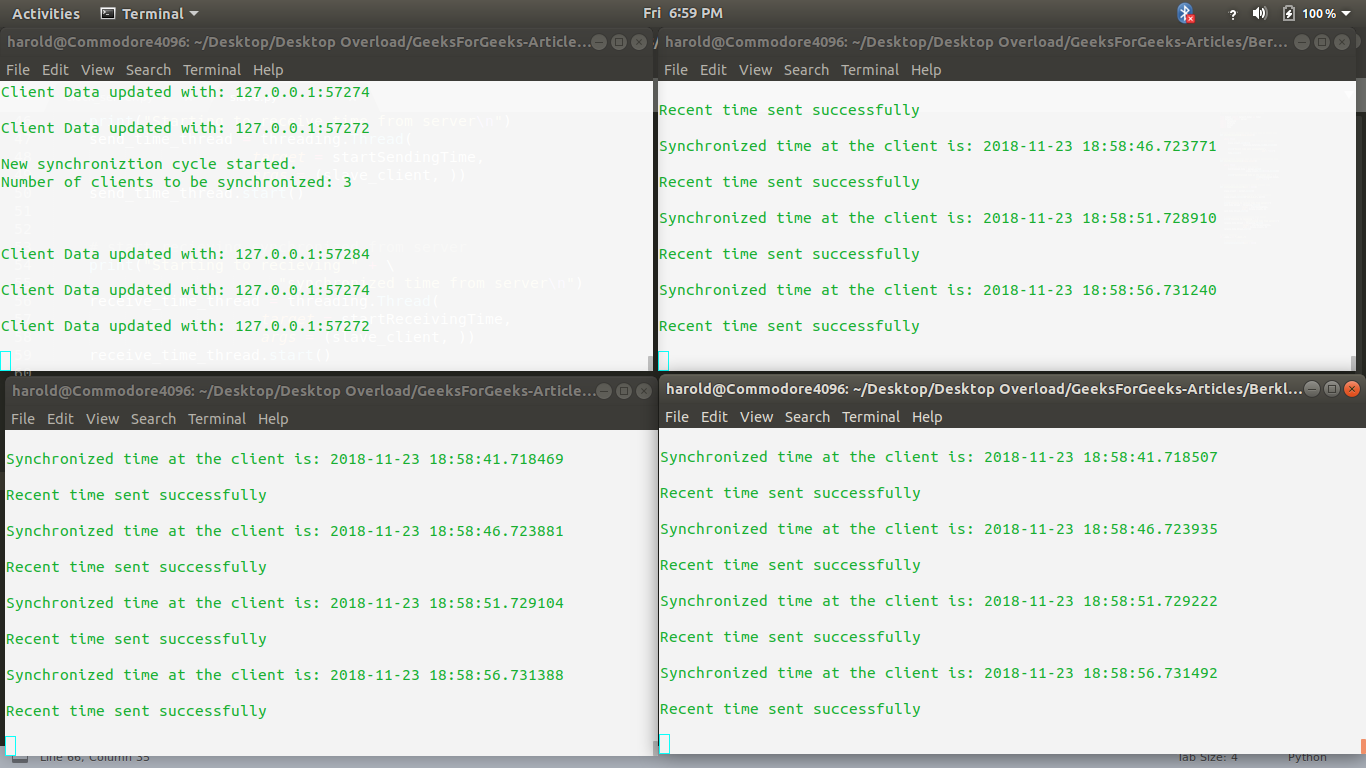Open in App
Not now

# Berkeley’s Algorithm

• Difficulty Level : Medium
• Last Updated : 15 Mar, 2023

Berkeley’s Algorithm is a clock synchronization technique used in distributed systems. The algorithm assumes that each machine node in the network either doesn’t have an accurate time source or doesn’t possess a UTC server.
Algorithm
1) An individual node is chosen as the master node from a pool node in the network. This node is the main node in the network which acts as a master and the rest of the nodes act as slaves. The master node is chosen using an election process/leader election algorithm.
2) Master node periodically pings slaves nodes and fetches clock time at them using Cristian’s algorithm.
The diagram below illustrates how the master sends requests to slave nodes.The diagram below illustrates how slave nodes send back time given by their system clock.3) Master node calculates the average time difference between all the clock times received and the clock time given by the master’s system clock itself. This average time difference is added to the current time at the master’s system clock and broadcasted over the network.
Pseudocode for above step:

` `

The diagram below illustrates the last step of Berkeley’s algorithm.Scope of Improvement

• Improvision inaccuracy of Cristian’s algorithm.
• Ignoring significant outliers in the calculation of average time difference
• In case the master node fails/corrupts, a secondary leader must be ready/pre-chosen to take the place of the master node to reduce downtime caused due to the master’s unavailability.
• Instead of sending the synchronized time, master broadcasts relative inverse time difference, which leads to a decrease in latency induced by traversal time in the network while the time of calculation at slave node.

### features of Berkeley’s Algorithm:

Centralized time coordinator: Berkeley’s Algorithm uses a centralized time coordinator, which is responsible for maintaining the global time and distributing it to all the client machines.

Clock adjustment: The algorithm adjusts the clock of each client machine based on the difference between its local time and the time received from the time coordinator.

Average calculation: The algorithm calculates the average time difference between the client machines and the time coordinator to reduce the effect of any clock drift.

Fault tolerance: Berkeley’s Algorithm is fault-tolerant, as it can handle failures in the network or the time coordinator by using backup time coordinators.

Accuracy: The algorithm provides accurate time synchronization across all the client machines, reducing the chances of errors due to time discrepancies.

Scalability: The algorithm is scalable, as it can handle a large number of client machines, and the time coordinator can be easily replicated to provide high availability.

Security: Berkeley’s Algorithm provides security mechanisms such as authentication and encryption to protect the time information from unauthorized access or tampering.

The code below is a python script that can be used to trigger a master clock server.

## Python3

 `# Python3 program imitating a clock server` `from` `functools ``import` `reduce``from` `dateutil ``import` `parser``import` `threading``import` `datetime``import` `socket``import` `time`  `# datastructure used to store client address and clock data``client_data ``=` `{}`  `''' nested thread function used to receive``    ``clock time from a connected client '''``def` `startReceivingClockTime(connector, address):` `    ``while` `True``:``        ``# receive clock time``        ``clock_time_string ``=` `connector.recv(``1024``).decode()``        ``clock_time ``=` `parser.parse(clock_time_string)``        ``clock_time_diff ``=` `datetime.datetime.now() ``-` `\``                                                 ``clock_time` `        ``client_data[address] ``=` `{``                       ``"clock_time"`      `: clock_time,``                       ``"time_difference"` `: clock_time_diff,``                       ``"connector"`       `: connector``                       ``}` `        ``print``(``"Client Data updated with: "``+` `str``(address),``                                              ``end ``=` `"\n\n"``)``        ``time.sleep(``5``)`  `''' master thread function used to open portal for``    ``accepting clients over given port '''``def` `startConnecting(master_server):``    ` `    ``# fetch clock time at slaves / clients``    ``while` `True``:``        ``# accepting a client / slave clock client``        ``master_slave_connector, addr ``=` `master_server.accept()``        ``slave_address ``=` `str``(addr[``0``]) ``+` `":"` `+` `str``(addr[``1``])` `        ``print``(slave_address ``+` `" got connected successfully"``)` `        ``current_thread ``=` `threading.Thread(``                         ``target ``=` `startReceivingClockTime,``                         ``args ``=` `(master_slave_connector,``                                           ``slave_address, ))``        ``current_thread.start()`  `# subroutine function used to fetch average clock difference``def` `getAverageClockDiff():` `    ``current_client_data ``=` `client_data.copy()` `    ``time_difference_list ``=` `list``(client[``'time_difference'``]``                                ``for` `client_addr, client``                                    ``in` `client_data.items())``                                   `  `    ``sum_of_clock_difference ``=` `sum``(time_difference_list, \``                                   ``datetime.timedelta(``0``, ``0``))` `    ``average_clock_difference ``=` `sum_of_clock_difference \``                                         ``/` `len``(client_data)` `    ``return`  `average_clock_difference`  `''' master sync thread function used to generate``    ``cycles of clock synchronization in the network '''``def` `synchronizeAllClocks():` `    ``while` `True``:` `        ``print``(``"New synchronization cycle started."``)``        ``print``(``"Number of clients to be synchronized: "` `+` `\``                                     ``str``(``len``(client_data)))` `        ``if` `len``(client_data) > ``0``:` `            ``average_clock_difference ``=` `getAverageClockDiff()` `            ``for` `client_addr, client ``in` `client_data.items():``                ``try``:``                    ``synchronized_time ``=` `\``                         ``datetime.datetime.now() ``+` `\``                                    ``average_clock_difference` `                    ``client[``'connector'``].send(``str``(``                               ``synchronized_time).encode())` `                ``except` `Exception as e:``                    ``print``(``"Something went wrong while "` `+` `\``                          ``"sending synchronized time "` `+` `\``                          ``"through "` `+` `str``(client_addr))` `        ``else` `:``            ``print``(``"No client data."` `+` `\``                        ``" Synchronization not applicable."``)` `        ``print``(``"\n\n"``)` `        ``time.sleep(``5``)`  `# function used to initiate the Clock Server / Master Node``def` `initiateClockServer(port ``=` `8080``):` `    ``master_server ``=` `socket.socket()``    ``master_server.setsockopt(socket.SOL_SOCKET,``                                   ``socket.SO_REUSEADDR, ``1``)` `    ``print``(``"Socket at master node created successfully\n"``)``      ` `    ``master_server.bind(('', port))` `    ``# Start listening to requests``    ``master_server.listen(``10``)``    ``print``(``"Clock server started...\n"``)` `    ``# start making connections``    ``print``(``"Starting to make connections...\n"``)``    ``master_thread ``=` `threading.Thread(``                        ``target ``=` `startConnecting,``                        ``args ``=` `(master_server, ))``    ``master_thread.start()` `    ``# start synchronization``    ``print``(``"Starting synchronization parallelly...\n"``)``    ``sync_thread ``=` `threading.Thread(``                          ``target ``=` `synchronizeAllClocks,``                          ``args ``=` `())``    ``sync_thread.start()`   `# Driver function``if` `__name__ ``=``=` `'__main__'``:` `    ``# Trigger the Clock Server``    ``initiateClockServer(port ``=` `8080``)`

Output:

```New synchronization cycle started.
Number of clients to be synchronized: 3

Client Data updated with: 127.0.0.1:57284

Client Data updated with: 127.0.0.1:57274

Client Data updated with: 127.0.0.1:57272```

The code below is a python script that can be used to trigger a slave/client.

## Python3

 `# Python3 program imitating a client process` `from` `timeit ``import` `default_timer as timer``from` `dateutil ``import` `parser``import` `threading``import` `datetime``import` `socket``import` `time`  `# client thread function used to send time at client side``def` `startSendingTime(slave_client):` `    ``while` `True``:``        ``# provide server with clock time at the client``        ``slave_client.send(``str``(``                       ``datetime.datetime.now()).encode())` `        ``print``(``"Recent time sent successfully"``,``                                          ``end ``=` `"\n\n"``)``        ``time.sleep(``5``)`  `# client thread function used to receive synchronized time``def` `startReceivingTime(slave_client):` `    ``while` `True``:``        ``# receive data from the server``        ``Synchronized_time ``=` `parser.parse(``                          ``slave_client.recv(``1024``).decode())` `        ``print``(``"Synchronized time at the client is: "` `+` `\``                                    ``str``(Synchronized_time),``                                    ``end ``=` `"\n\n"``)`  `# function used to Synchronize client process time``def` `initiateSlaveClient(port ``=` `8080``):` `    ``slave_client ``=` `socket.socket()         ``      ` `    ``# connect to the clock server on local computer``    ``slave_client.connect((``'127.0.0.1'``, port))` `    ``# start sending time to server``    ``print``(``"Starting to receive time from server\n"``)``    ``send_time_thread ``=` `threading.Thread(``                      ``target ``=` `startSendingTime,``                      ``args ``=` `(slave_client, ))``    ``send_time_thread.start()`  `    ``# start receiving synchronized from server``    ``print``(``"Starting to receiving "` `+` `\``                         ``"synchronized time from server\n"``)``    ``receive_time_thread ``=` `threading.Thread(``                       ``target ``=` `startReceivingTime,``                       ``args ``=` `(slave_client, ))``    ``receive_time_thread.start()`  `# Driver function``if` `__name__ ``=``=` `'__main__'``:` `    ``# initialize the Slave / Client``    ``initiateSlaveClient(port ``=` `8080``)`

Output:

```Recent time sent successfully
Synchronized time at the client is: 2018-11-23 18:49:31.166449```

Below is a screenshot of the runtime of the above python scripts where the top left console represents the master thread while others represent slave threads.Note: The scripts above closely depicts working of Berkley’s Algorithm but may differ from the actual implementation of the algorithm in production based distributed networking systems. Availability of port 8080 is machine dependent. In case port 8080 is not free, change the port number accordingly in both master and slave scripts.

References:

My Personal Notes arrow_drop_up